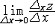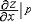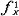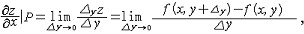1)  Boolean partial derivative1.
New method testing the double fault of combinational circuits based on Boolean partial derivative.;

2.
Tabular method of calculating Boolean partial derivative and difference of the OC type logic function.;

3.
Methods of calculating Boolean partial derivative based on d_j-map.;2)  Boolean polarization-encoded logic algebra1.
In this paper,the Boolean polarization-encoded logic algebra(BPLA)is used to designoptical parallel array logic gates and liquid crystal encoded ripple-carry full adder.

3)  Partial derivative1.
A Principle of calculus partial derivative of homogenous polynomial of degree m;2.
A Definition of Partial Derivative of Random Functions and Its Application to RBFNN Sensitivity Analysis;

3.
Modified method for matrix orthogonalization and its partial derivative4)  e-partial derivativee-偏导数
1.
In order to discuss the properties of Bent Function based on e-partial derivative and relationship of Bent Function and linear Function,the paper proposed a relatively simple algorithm no matter whether the Boolean Function is Bent Function or not.

5)  partial derivative method1.
A computational scheme is presented to evaluate the dynamic stiffness and damping coefficients of the aerodynamic tilting-pad journal bearings by combining the partial derivative method with the equivalent coefficient method.

2.
A novel and universal computational method for obtaining the dynamic stiffness and dynamic damping coefficients of aerodynamic bearings is presented by means of the partial derivative method applied to the gas-lubricated Reynolds equation,and the dynamic stiffness and dynamic damping coefficients of a typical aerodynamic bearing are eva.

6)  partial derivatives1.
We are familiar with the algorithm of the partial derivatives from the calculus.2.
This paper presents the method of designing active filters in which the first and second-order partial derivatives of the transfer function with respect to the time constant of the OA s vanish.

3.
The filters are based on local partial derivatives.偏导数partial derivative    二元函数z＝f（x，y）沿坐标轴方向的方向导数（或沿坐标轴方向的变化率）。即把z＝f（x，y）中的一个自变量y看作常数，于是z＝f（x，y）就成为关于x的一元函数，给x以改变量Δx，则有z关于x的（偏）改变量Δxz＝f（x＋Δx，y）－f（x，y），如果极限(存在且有限，就称此极限为二元函数 z ＝f（ x，y ）在 P（x，y）点关于x的偏导数，记作，或（x，y），类似地有说明：补充资料仅用于学习参考，请勿用于其它任何用途。 参考词条 ©2011 dictall.com